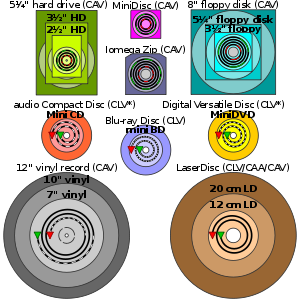# Constant angular velocity

﻿
Constant angular velocityComparison of several forms of disk storage showing tracks (not-to-scale); green denotes start and red denotes end.
* Some CD-R(W) and DVD-R(W)/DVD+R(W) recorders operate in ZCLV, CAA or CAV modes.

In optical storage, constant angular velocity (CAV) is a qualifier for the rated speed of an optical disc drive, and may also be applied to the writing speed of recordable discs. A drive or disc operating in CAV mode maintains a constant angular velocity, contrasted with a constant linear velocity (CLV).

Like all spinning-disk media, the CD-ROM drive includes a spindle motor that turns the media containing the data to be read. The spindle motor of a standard CD-ROM is very different from that of a hard disk or floppy drive in one very important way: it does not spin at a constant speed. Rather, the speed of the drive varies depending on what part of the disk (inside vs. outside) is being read.

Standard hard disks and floppy disks spin the disk at a constant speed. Regardless of where the heads are, the same speed is used to turn the media. This is called constant angular velocity (CAV) because it takes the same amount of time for a turn of the 360 degrees of the disk at all times. Since the tracks on the inside of the disk are much smaller than those on the outside of the disk, this constant speed means that when the heads are on the outside of the disk they will traverse a much longer linear path than they do when on the inside. Hence, the linear velocity is not constant.

Some high speed CD and DVD drives use CAV.

CAV was used in the LaserDisc format for interactive titles, as well as special editions of certain films. CAV allowed for perfect still frames, as well as random access to any given frame on a disc. Playing time, however, was cut in half from 60 minutes to 30 minutes.

CAV is used with Nintendo GameCube Game Disc and Wii Optical Disc, as well as the Sega GD-rom.

Wikimedia Foundation. 2010.

### Look at other dictionaries:

• Constant Angular Velocity — Constant Angular Velocity,   CAV Verfahren …   Universal-Lexikon

• constant angular velocity —    Abbreviated CAV. An unchanging speed of rotation. Hard disks use a CAV encoding scheme. The constant rate of rotation means that sectors on the disk are at the maximum density along the inside track of the disk. As the read/write heads move… …   Dictionary of networking

• Constant Angular Velocity — CAV, revolution speed of a hard disk that does not change according to the location of data on the disk (as opposed to CLV in which the speed is faster on the inner tracks) …   English contemporary dictionary

• Constant linear velocity — Comparison of several forms of disk storage showing tracks (not to scale); green denotes start and red denotes end. * Some CD R(W) and DVD R(W)/DVD+R(W) recorders operate in ZCLV, CAA or CAV modes. In optical storage, constant linear velocity… …   Wikipedia

• constant linear velocity —    Abbreviated CLV. A changing speed of rotation. CDROM disk drives use a CLV encoding scheme to make sure that the data density remains constant. Information on a compact disc is stored in a single, spiral track, divided into many equal length… …   Dictionary of networking

• Constant-velocity joint — Rzeppa six ball CV joint Constant velocity joints (aka homokinetic or CV joints) allow a drive shaft to transmit power through a variable angle, at constant rotational speed, without an appreciable increase in friction or play. They are mainly… …   Wikipedia

• Angular acceleration — is the rate of change of angular velocity over time. In SI units, it is measured in radians per second squared (rad/s2), and is usually denoted by the Greek letter alpha ({alpha},). Mathematical definition The angular acceleration can be defined… …   Wikipedia

• Angular displacement — of a body is the angle in radians (degrees, revolutions) through which a point or line has been rotated in a specified sense about a specified axis.When an object rotates about its axis, the motion cannot simply be analyzed as a particle, since… …   Wikipedia

• Angular momentum — For a generally accessible and less technical introduction to the topic, see Introduction to angular momentum. Classical mechanics Newton s Second Law …   Wikipedia

• Velocity — In physics, velocity is defined as the rate of change of position. It is a vector physical quantity; both speed and direction are required to define it. In the SI (metric) system, it is measured in meters per second: (m/s) or ms 1. The scalar… …   Wikipedia